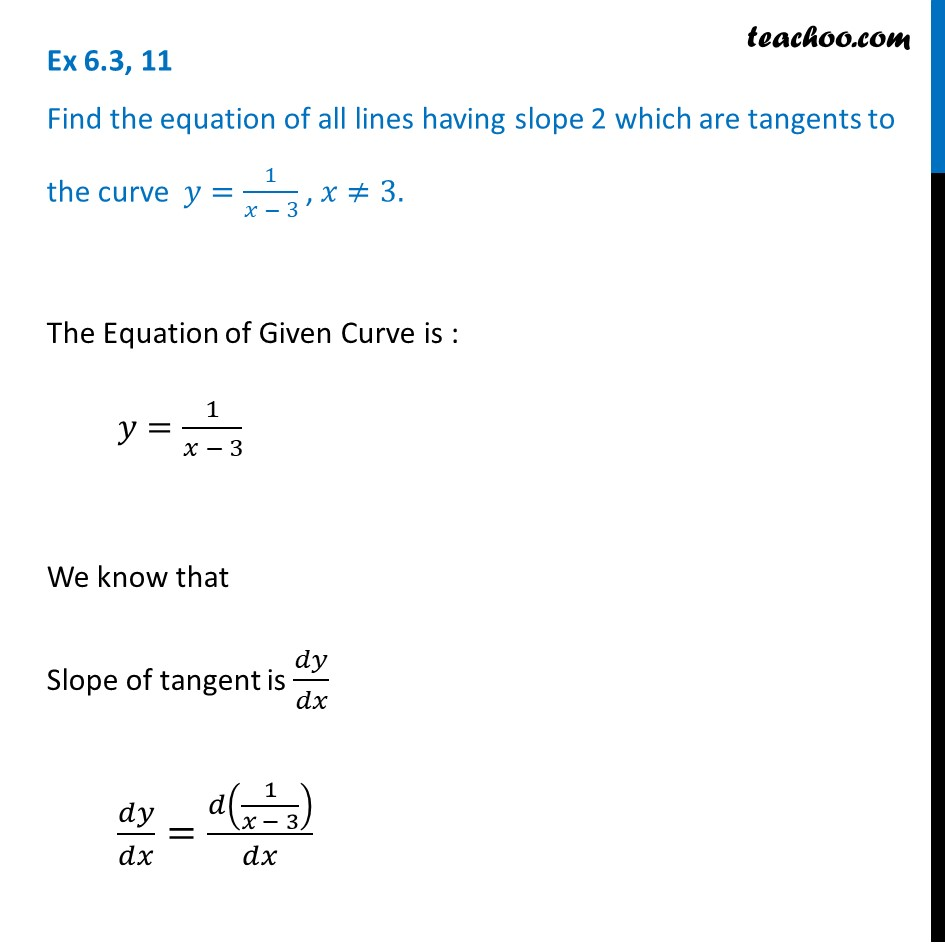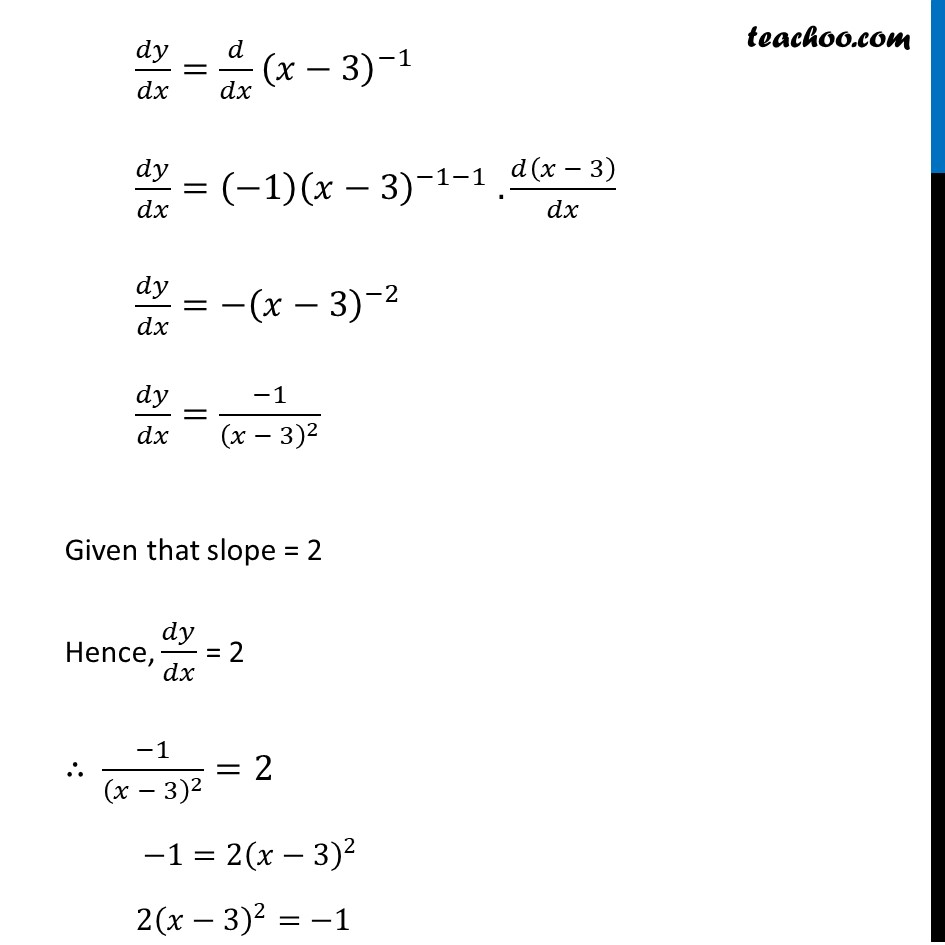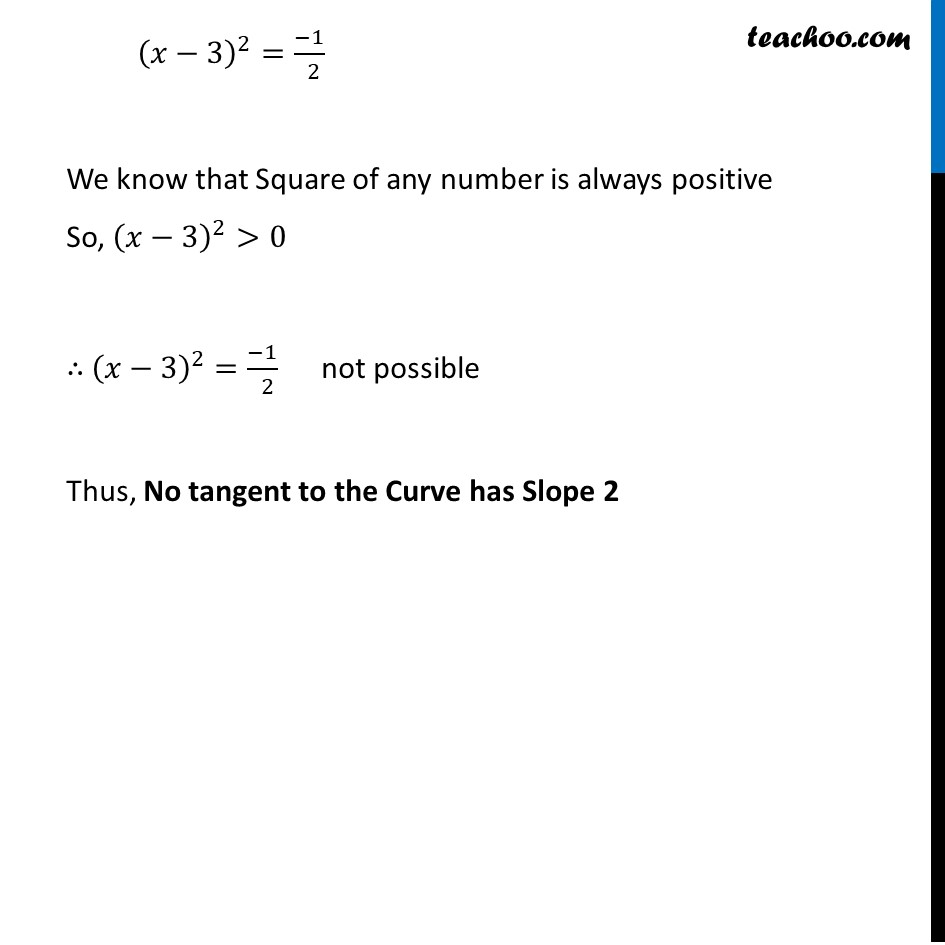Tangents and Normals (using Differentiation)

Chapter 6 Class 12 Application of Derivatives
Serial order wiseLearn in your speed, with individual attention - Teachoo Maths 1-on-1 Class

### Transcript

Question 11 Find the equation of all lines having slope 2 which are tangents to the curve 𝑦=1/(𝑥 − 3) , 𝑥≠3.The Equation of Given Curve is : 𝑦=1/(𝑥 − 3) We know that Slope of tangent is 𝑑𝑦/𝑑𝑥 𝑑𝑦/𝑑𝑥=𝑑(1/(𝑥 − 3))/𝑑𝑥 𝑑𝑦/𝑑𝑥=𝑑/𝑑𝑥 (𝑥−3)^(−1) 𝑑𝑦/𝑑𝑥=(−1) (𝑥−3)^(−1−1) . 𝑑(𝑥 − 3)/𝑑𝑥 𝑑𝑦/𝑑𝑥=−(𝑥−3)^(−2) 𝑑𝑦/𝑑𝑥=(−1)/(𝑥 − 3)^2 Given that slope = 2 Hence, 𝑑𝑦/𝑑𝑥 = 2 ∴ (−1)/(𝑥 − 3)^2 =2 −1=2(𝑥−3)^2 〖2(𝑥−3)〗^2=−1 (𝑥−3)^2=(−1)/( 2) We know that Square of any number is always positive So, (𝑥−3)^2>0 ∴ (𝑥−3)^2=(−1)/( 2) not possible Thus, No tangent to the Curve has Slope 2